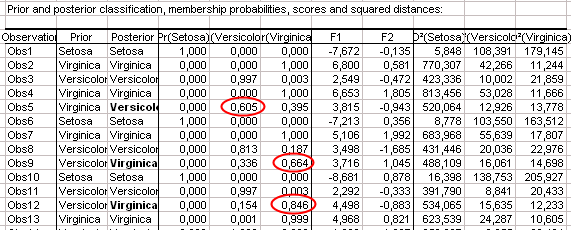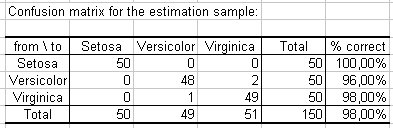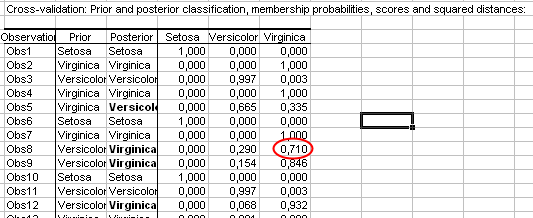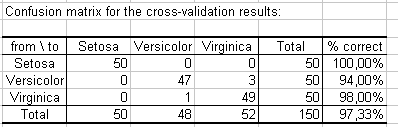Your data analysis solution

# Discriminant Analysis in Excel tutorial

2017-12-19

This tutorial will help you set up and interpret a Discriminant Analysis (DA) in Excel using the XLSTAT software.

## Dataset for running a Discriminant Analysis

An Excel sheet with both the data and the results can be downloaded by clicking on the button below:

The data are from [Fisher M. (1936). The Use of Multiple Measurements in Taxonomic Problems. Annals of Eugenics, 7, 179 -188] and correspond to 150 Iris flowers, described by four variables (sepal length, sepal width, petal length, petal width) and their species. Three different species have been included in this study: setosa, versicolor and virginica.

## Goal of this Discriminant Analysis

Our goal is to test if the four variables allow to discriminate the species, and to visualize the observations on a 2-dimensional map that shows as well as possible how separated the groups are.Iris setosa, versicolor and virginica.

## Setting up a Discriminant Analysis

After opening XLSTAT, select the XLSTAT / Analyzing data / Discriminant analysis command, or click on the corresponding button of the Analyzing data toolbar (see below).Once you've clicked on the button, the Discriminant analysis dialog box appears. The qualitative dependent variable corresponds here to the "Species" variable. The quantitative Explanatory variables are the four descriptive variables.We uncheck the Equality of covariance matrices option because, as we will see with the Box's test, assuming that the covariance matrices of the three species are equal would be wrong.Many results are optionally displayed by XLSTAT. We can see below which options have been activated for this particular case.In order to avoid adding too much information on the plots, we have unchecked the Labels option in the Charts tab.The computations begin once you have clicked on OK. The results will then be displayed.

## Interpreting the results of a Discriminant Analysis

The first results displayed are the various matrices used for the computations. The two Box's tests confirm that we need to reject the hypothesis that the covariance matrices are equal between the groups.The Wilks' Lambda test allows to test if the vector of the means for the various groups are equal or not (you can understand it as a multidimensional version of the Fisher's LSD or the Tukey's HSD tests). We see that the difference between the means vectors of the groups is significant.The next table shows the eigenvalues and the corresponding % of variance. We can see that 99% of the variance is represented with the first factor. There are only two factors: the maximum number of factors is equal to k-1, when n>p>k, where n is the number of observations, p the number of explanatory variables, and k the number of groups.The following chart shows how the initial variables are correlated with the two factors (this chart corresponds to the factor loadings table). We can see that the factor F1 is correlated with Sepal length, Petal length, and Petal width and that F2 is correlated with Sepal width.The following table displays the discriminant functions. When we assume the equality of the covariance matrices, the discriminant functions are linear. When the equality is not assumed, which is the case in this tutorial, the discriminant functions are quadratic. The rule based on these functions is that we allocate an observation to the group corresponding to the function that gives the greatest value. These functions can be used in predictive mode on new observations to allocate them to a group.The next table lists for each observation the factor scores (the coordinates of the observations in the new space), the probability to belong to each group, and the squared Mahalanobis distances to the centroid of the group. Each observation is classified into the group for the which the probability of belonging is the greatest. The probabilities are posterior probabilities that take into account the prior probabilities through the Bayes formula. We notice that three observations (5,9,12) have been reclassified. There are several ways in which these results can be interpreted: either the person who made the measures made an error when recording the values, or the corresponding iris flowers have had a very unusual growth or the criteria used by the specialist to determine the species was not precise enough, or some information necessary to discrimine the flowers is not available here.The following chart represents the observations on the factor axes. It allows to confirm that the species are very well discriminated on the factor axes extracted from the original explanatory variables.The confusion matrix summarizes the reclassification of the observations, and allows to quickly see the % of well classified observations, which is the ratio of the number of observations that have been well classified over the total number of observations. It is here equal to 98%.As the corresponding option has been activated in the "Outputs" tab of the dialog box, the predictions for the cross-validation are computed. Cross-validation allows to see what would be the prediction for a given observation if it is left out of the estimation sample. We can see here that only one more observation (Obs8) is miss-classified.The confusion matrix of the cross-validation is displayed below.### Contact Us

#### Contact our technical support team: support@xlstat.com

https://cdn.desk.com/
false
desk
Loading
seconds ago
a minute ago
minutes ago
an hour ago
hours ago
a day ago
days ago
about
false
Invalid characters found
/customer/portal/articles/autocomplete
9283